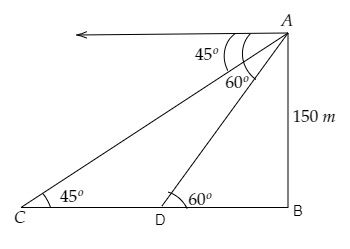# On the same side of a tower, two objects are located. When observed from the top of the tower, their angles of depression are $45^{\circ}$ and $60^{\circ} .$ If the height of the tower is $150 \mathrm{~m}$, find the distance between the objects.

Given:

On the same side of a tower, two objects are located. When observed from the top of the tower, their angles of depression are $45^{\circ}$ and $60^{\circ} .$

The height of the tower is $150 \mathrm{~m}$.

To do:

We have to find the distance between the objects.

Solution:Let $AB$ be the height of the tower and $C, D$ be the points where the two objects are placed whose angles of depression are $45^{\circ}$ and $60^{\circ}$ respectively.

From the figure,

$\mathrm{AB}=150 \mathrm{~m}, \angle \mathrm{ACB}=45^{\circ}, \angle \mathrm{ADB}=60^{\circ}$       (Since, alternate angles are equal)

Let the object $C$ be at a distance of $\mathrm{CB}=x \mathrm{~m}$ from the foot of the tower and the distance between the points $C$ and $D$ be $\mathrm{CD}=y \mathrm{~m}$.

This implies,

$\mathrm{CB}=x-y \mathrm{~m}$

We know that,

$\tan \theta=\frac{\text { Opposite }}{\text { Adjacent }}$

$=\frac{\text { AB }}{BC}$

$\Rightarrow \tan 45^{\circ}=\frac{150}{x}$

$\Rightarrow 1=\frac{150}{x}$

$\Rightarrow x=150 \mathrm{~m}$..........(i)

Similarly,

$\tan \theta=\frac{\text { Opposite }}{\text { Adjacent }}$

$=\frac{\text { AB }}{DB}$

$\Rightarrow \tan 60^{\circ}=\frac{150}{x-y}$

$\Rightarrow \sqrt3=\frac{150}{150-y}$

$\Rightarrow (150-y)\sqrt3=150 \mathrm{~m}$

$\Rightarrow 150\sqrt3-150=y\sqrt3 \mathrm{~m}$           [From (i)]

$\Rightarrow y=\frac{150(1.73)-150}{\sqrt3} \mathrm{~m}$

$\Rightarrow y=\frac{150(1.732-1)}{1.732} \mathrm{~m}$

$\Rightarrow y=\frac{150\times0.732}{1.732} \mathrm{~m}$

$\Rightarrow y=\frac{109.8}{1.732}=63.4 \mathrm{~m}$

Therefore, the distance between the two objects is $63.4 \mathrm{~m}$.

Updated on: 10-Oct-2022

41 Views